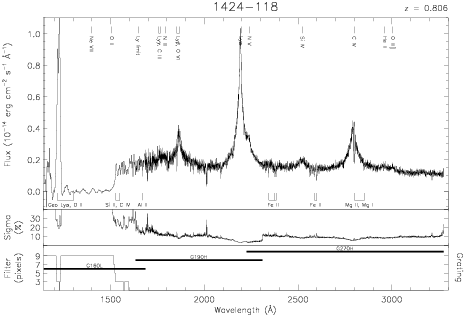Download dataset
TELESCOP= 'HST     '
INSTRUME= 'FOS     '
EQUINOX =               2000.0
DATE-OBS= '1992-03-13T13:50:27'
RA_PNT  =       216.9087500000
DEC_PNT =       -12.0638611111
PA_PNT  =        28.9925247155
OBJECT  = '1424-118'
ROOTNM01= 'Y0RV1503T'
DETECT01= 'AMBER   '
APERID01= 'C-2     '
FGWAID01= 'H19     '
ROOTNM02= 'Y0RV1504T'
DETECT02= 'AMBER   '
APERID02= 'C-2     '
FGWAID02= 'H19     '
ROOTNM03= 'Y0RV1505T'
DETECT03= 'AMBER   '
APERID03= 'C-2     '
FGWAID03= 'H19     '
ROOTNM04= 'Y0RV1506T'